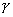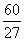Thursday 04th June 2020
 CBSE Guess > Papers > Question Papers > Class XII > 2005 > Chemistry > Delhi Set-III CHEMISTRY 2005 (Set-III Delhi)
Except for the following questions, all the remaining questions have been asked in Set I and Set II. Q. 12. Write the names and structures of the monomers used for getting the following polymers: 2 (i) PVC (ii) PMMA Q. 18. What are the two classes of emulsions? Give one example of each class State one activity to test the type of an emulsion. 3 Q. 23. The radioactive isotope Co can be made by both an (n, p) and an (n,) reaction For each reaction indicate the appropriate target nucleus If the half-life ofCo is 7 years, calculate the dacay constant for it. 3 Q. 24. What are carbon fibres and what properties make them specially useful? Write two uses of carbon fibres. 3 Q. 26. (a) Assign a reason for each of the following statements: (i) Unlike In+ TI+ is stable with respect to disproportionation reaction: (ii) Pérehioric acid is a stronger acid than sulphuric acid. (iii) Of all the noble gases only Xenon is known to form established chemi cal compounds. (b) Write the structural formulae for the following: 3, 2 (i) SF4 (ii) XeO3 Or  (a) Assign a reason for each of the following statements: (i) Anhydrous AICI3 acts as a catalyst in many organic reactions. (ii) All the bonds in a molecule of PCI5 are not equal. (iii) Hydrogen fluoride is a weaker acid than hydrogen chloride in aqueous solution. (b) Write structure of the following molecules: (i) IF5 (ii) XeOF3 Q. 27. (a) How are the lipids classified? Give one example of each class. (b) Name the deficiency diseases resulting from lack of Vitamins A and E in the diet. 5 Or (a) Define vitamins and state their classification. List two vitamins of each class. (b) What are enzymes? State the activity of an enzyme. English 2005 Question Papers Class XII Delhi Outside Delhi Set I Set I Set II Set II Set III Set III CBSE 2005 Question Papers Class XII